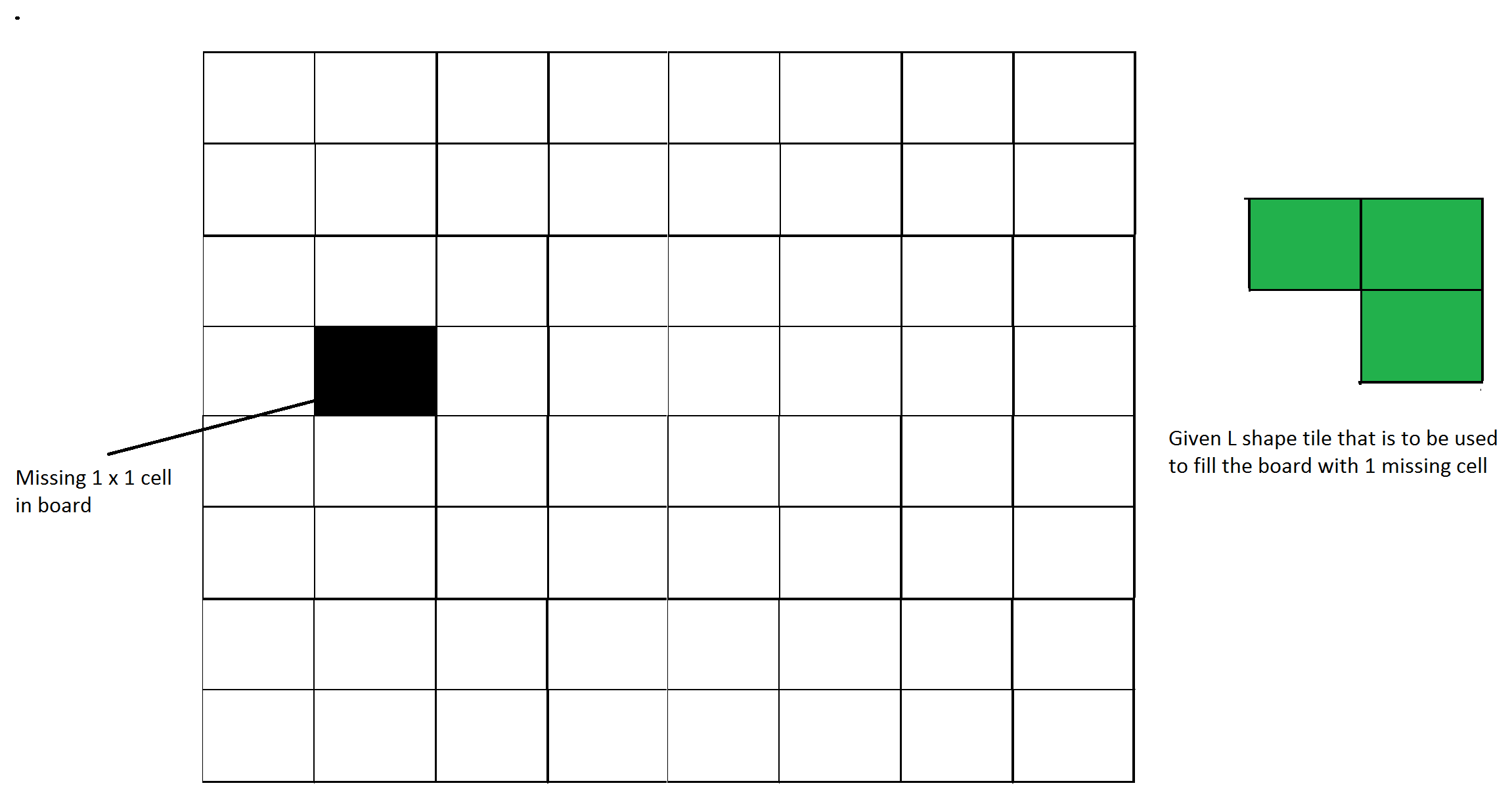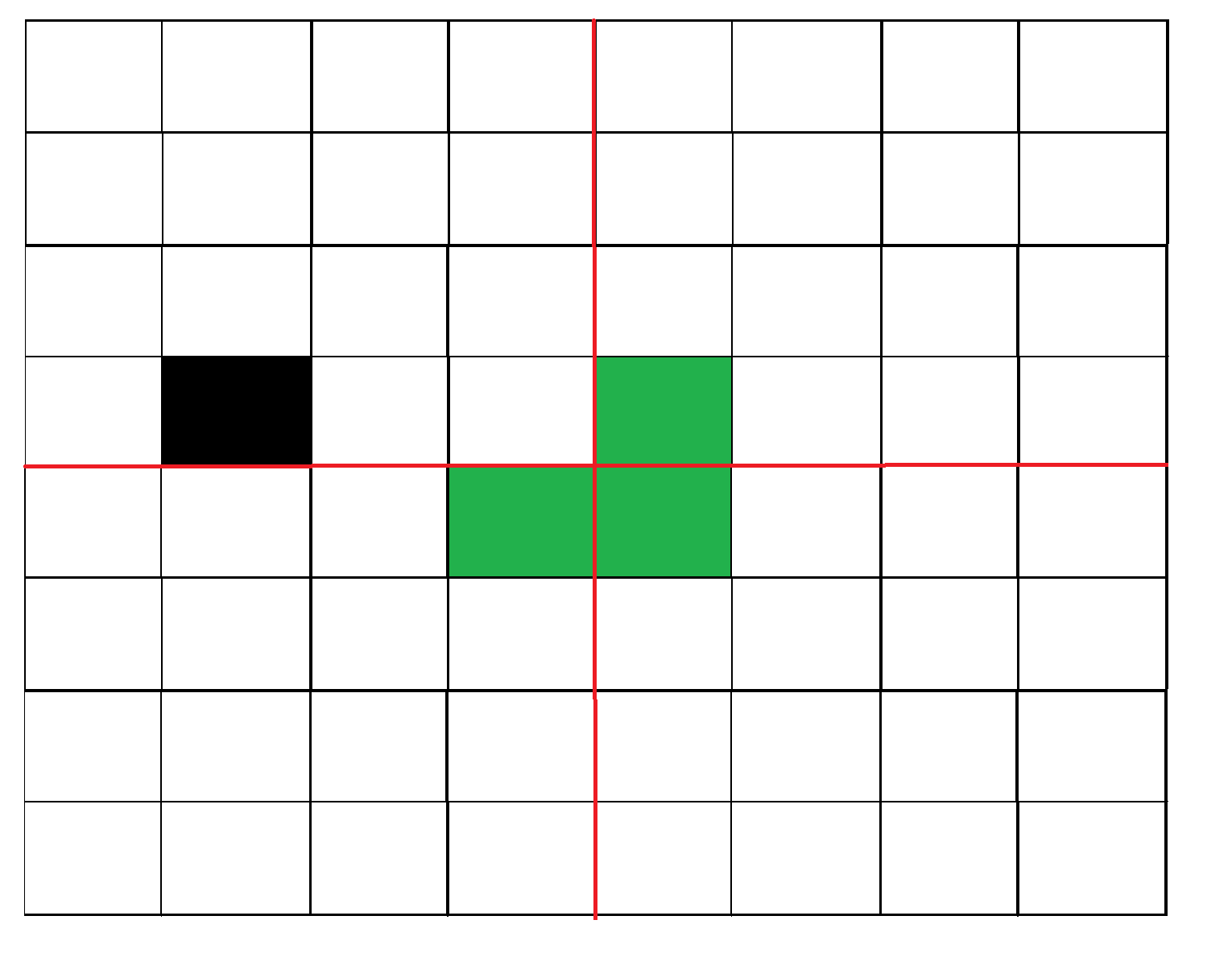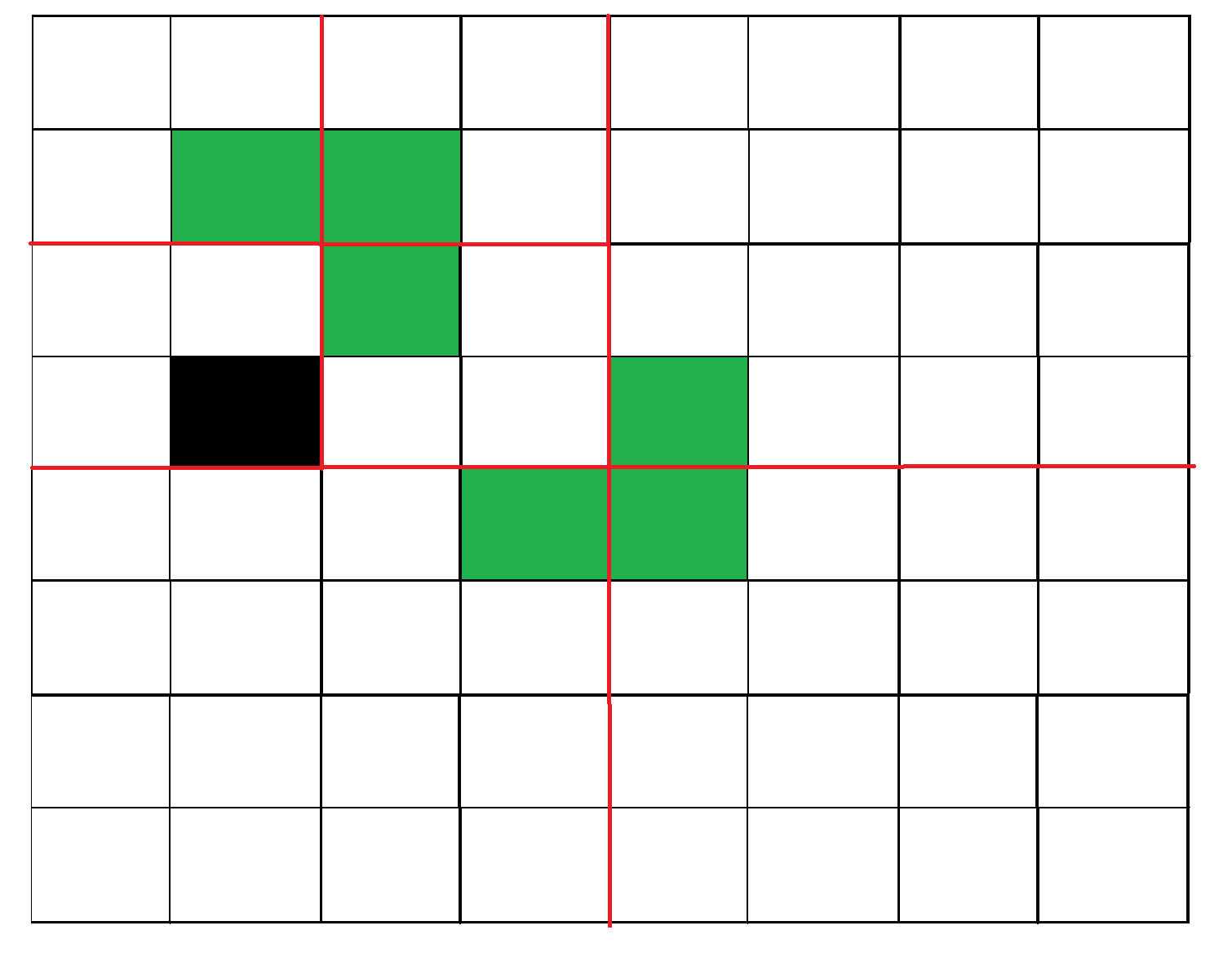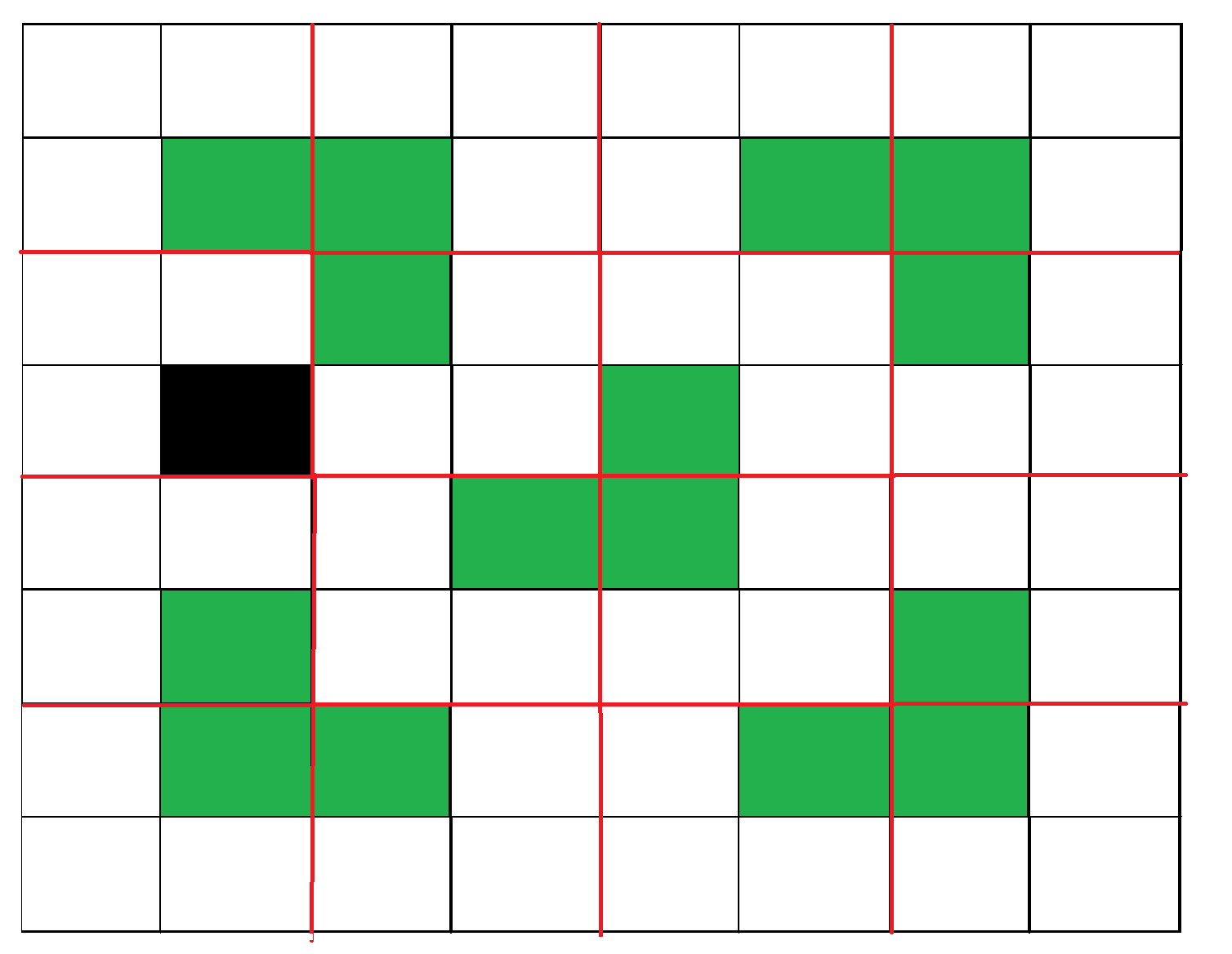# Tiling Problem using Divide and Conquer algorithm

• Difficulty Level : Hard
• Last Updated : 03 Feb, 2021

Given a n by n board where n is of form 2k where k >= 1 (Basically n is a power of 2 with minimum value as 2). The board has one missing cell (of size 1 x 1). Fill the board using L shaped tiles. A L shaped tile is a 2 x 2 square with one cell of size 1×1 missing.Attention reader! Don’t stop learning now. Get hold of all the important DSA concepts with the DSA Self Paced Course at a student-friendly price and become industry ready.  To complete your preparation from learning a language to DS Algo and many more,  please refer Complete Interview Preparation Course.

In case you wish to attend live classes with experts, please refer DSA Live Classes for Working Professionals and Competitive Programming Live for Students.

Figure 1: An example input
This problem can be solved using Divide and Conquer. Below is the recursive algorithm.

```// n is size of given square, p is location of missing cell
Tile(int n, Point p)

1) Base case: n = 2, A 2 x 2 square with one cell missing is nothing
but a tile and can be filled with a single tile.

2) Place a L shaped tile at the center such that it does not cover
the n/2 * n/2 subsquare that has a missing square. Now all four
subsquares of size n/2 x n/2 have a missing cell (a cell that doesn't
need to be filled).  See figure 2 below.

3) Solve the problem recursively for following four. Let p1, p2, p3 and
p4 be positions of the 4 missing cells in 4 squares.
a) Tile(n/2, p1)
b) Tile(n/2, p2)
c) Tile(n/2, p3)
d) Tile(n/2, p3)```

The below diagrams show working of above algorithmFigure 2: After placing first tileFigure 3: Recurring for first subsquare.Figure 4: Shows first step in all four subsquares.

Examples:

```Input :  size = 2 and mark coordinates = (0, 0)
Output :
-1      1
1       1
Coordinate (0, 0) is marked. So, no tile is there. In the remaining three positions,
a tile is placed with its number as 1.
Input : size = 4 and mark coordinates = (0, 0)
Output :
-1      3       2       2
3       3       1       2
4       1       1       5
4       4       5       5```

Below is C++ implementation of above idea:

## CPP

 `// C++ program to place tiles``#include ``using` `namespace` `std;` `int` `size_of_grid, b, a, cnt = 0;``int` `arr;` `// Placing tile at the given coordinates``void` `place(``int` `x1, ``int` `y1, ``int` `x2,``           ``int` `y2, ``int` `x3, ``int` `y3)``{``    ``cnt++;``    ``arr[x1][y1] = cnt;``    ``arr[x2][y2] = cnt;``    ``arr[x3][y3] = cnt;``}``// Quadrant names``// 1   2``// 3   4` `// Function based on divide and conquer``int` `tile(``int` `n, ``int` `x, ``int` `y)``{``    ``int` `r, c;``    ``if` `(n == 2) {``        ``cnt++;``        ``for` `(``int` `i = 0; i < n; i++) {``            ``for` `(``int` `j = 0; j < n; j++) {``                ``if` `(arr[x + i][y + j] == 0) {``                    ``arr[x + i][y + j] = cnt;``                ``}``            ``}``        ``}``        ``return` `0;``    ``}``    ``// finding hole location``    ``for` `(``int` `i = x; i < x + n; i++) {``        ``for` `(``int` `j = y; j < y + n; j++) {``            ``if` `(arr[i][j] != 0)``                ``r = i, c = j;``        ``}``    ``}` `    ``// If missing tile is 1st quadrant``    ``if` `(r < x + n / 2 && c < y + n / 2)``        ``place(x + n / 2, y + (n / 2) - 1, x + n / 2,``              ``y + n / 2, x + n / 2 - 1, y + n / 2);` `    ``// If missing Tile is in 3rd quadrant``    ``else` `if` `(r >= x + n / 2 && c < y + n / 2)``        ``place(x + (n / 2) - 1, y + (n / 2), x + (n / 2),``              ``y + n / 2, x + (n / 2) - 1, y + (n / 2) - 1);` `    ``// If missing Tile is in 2nd quadrant``    ``else` `if` `(r < x + n / 2 && c >= y + n / 2)``        ``place(x + n / 2, y + (n / 2) - 1, x + n / 2,``              ``y + n / 2, x + n / 2 - 1, y + n / 2 - 1);` `    ``// If missing Tile is in 4th quadrant``    ``else` `if` `(r >= x + n / 2 && c >= y + n / 2)``        ``place(x + (n / 2) - 1, y + (n / 2), x + (n / 2),``              ``y + (n / 2) - 1, x + (n / 2) - 1,``              ``y + (n / 2) - 1);` `    ``// diving it again in 4 quadrants``    ``tile(n / 2, x, y + n / 2);``    ``tile(n / 2, x, y);``    ``tile(n / 2, x + n / 2, y);``    ``tile(n / 2, x + n / 2, y + n / 2);` `    ``return` `0;``}``// Driver program to test above function``int` `main()``{``    ``// size of box``    ``size_of_grid = 8;``    ``memset``(arr, 0, ``sizeof``(arr));``    ``// Coordinates which will be marked``    ``a = 0, b = 0;``    ``// Here tile can not be placed``    ``arr[a][b] = -1;``    ``tile(size_of_grid, 0, 0);``    ``// The grid is``    ``for` `(``int` `i = 0; i < size_of_grid; i++) {``        ``for` `(``int` `j = 0; j < size_of_grid; j++)``            ``cout << arr[i][j] << ``" \t"``;``        ``cout << ``"\n"``;``    ``}``}`

## Java

 `// Java program to place tiles``public` `class` `GFG``{``  ``static` `int` `size_of_grid, b, a, cnt = ``0``;``  ``static` `int``[][] arr = ``new` `int``[``128``][``128``];` `  ``// Placing tile at the given coordinates``  ``static` `void` `place(``int` `x1, ``int` `y1, ``int` `x2,``                    ``int` `y2, ``int` `x3, ``int` `y3)``  ``{``    ``cnt++;``    ``arr[x1][y1] = cnt;``    ``arr[x2][y2] = cnt;``    ``arr[x3][y3] = cnt;``  ``}``  ``// Quadrant names``  ``// 1   2``  ``// 3   4` `  ``// Function based on divide and conquer``  ``static` `int` `tile(``int` `n, ``int` `x, ``int` `y)``  ``{``    ``int` `r = ``0``, c = ``0``;``    ``if` `(n == ``2``)``    ``{``      ``cnt++;``      ``for` `(``int` `i = ``0``; i < n; i++)``      ``{``        ``for` `(``int` `j = ``0``; j < n; j++)``        ``{``          ``if` `(arr[x + i][y + j] == ``0``)``          ``{``            ``arr[x + i][y + j] = cnt;``          ``}``        ``}``      ``}``      ``return` `0``;``    ``}` `    ``// finding hole location``    ``for` `(``int` `i = x; i < x + n; i++)``    ``{``      ``for` `(``int` `j = y; j < y + n; j++)``      ``{``        ``if` `(arr[i][j] != ``0``)``        ``{``          ``r = i;``          ``c = j;``        ``}` `      ``}``    ``}` `    ``// If missing tile is 1st quadrant``    ``if` `(r < x + n / ``2` `&& c < y + n / ``2``)``      ``place(x + n / ``2``, y + (n / ``2``) - ``1``, x + n / ``2``,``            ``y + n / ``2``, x + n / ``2` `- ``1``, y + n / ``2``);` `    ``// If missing Tile is in 3rd quadrant``    ``else` `if` `(r >= x + n / ``2` `&& c < y + n / ``2``)``      ``place(x + (n / ``2``) - ``1``, y + (n / ``2``), x + (n / ``2``),``            ``y + n / ``2``, x + (n / ``2``) - ``1``, y + (n / ``2``) - ``1``);` `    ``// If missing Tile is in 2nd quadrant``    ``else` `if` `(r < x + n / ``2` `&& c >= y + n / ``2``)``      ``place(x + n / ``2``, y + (n / ``2``) - ``1``, x + n / ``2``,``            ``y + n / ``2``, x + n / ``2` `- ``1``, y + n / ``2` `- ``1``);` `    ``// If missing Tile is in 4th quadrant``    ``else` `if` `(r >= x + n / ``2` `&& c >= y + n / ``2``)``      ``place(x + (n / ``2``) - ``1``, y + (n / ``2``), x + (n / ``2``),``            ``y + (n / ``2``) - ``1``, x + (n / ``2``) - ``1``,``            ``y + (n / ``2``) - ``1``);` `    ``// diving it again in 4 quadrants``    ``tile(n / ``2``, x, y + n / ``2``);``    ``tile(n / ``2``, x, y);``    ``tile(n / ``2``, x + n / ``2``, y);``    ``tile(n / ``2``, x + n / ``2``, y + n / ``2``); ``    ``return` `0``;``  ``}` `  ``// Driver code``  ``public` `static` `void` `main(String[] args)``  ``{` `    ``// size of box``    ``size_of_grid = ``8``;` `    ``// Coordinates which will be marked``    ``a = ``0``; b = ``0``;` `    ``// Here tile can not be placed``    ``arr[a][b] = -``1``;``    ``tile(size_of_grid, ``0``, ``0``);` `    ``// The grid is``    ``for` `(``int` `i = ``0``; i < size_of_grid; i++)``    ``{``      ``for` `(``int` `j = ``0``; j < size_of_grid; j++)``        ``System.out.print(arr[i][j] + ``" "``);``      ``System.out.println();;``    ``}``  ``}``}` `// This code is contributed by divyeshrabadiya07.`

## C#

 `// C# program to place tiles``using` `System;``class` `GFG``{``    ` `    ``static` `int` `size_of_grid, b, a, cnt = 0;``    ``static` `int``[,] arr = ``new` `int``[128, 128];``     ` `    ``// Placing tile at the given coordinates``    ``static` `void` `place(``int` `x1, ``int` `y1, ``int` `x2,``               ``int` `y2, ``int` `x3, ``int` `y3)``    ``{``        ``cnt++;``        ``arr[x1, y1] = cnt;``        ``arr[x2, y2] = cnt;``        ``arr[x3, y3] = cnt;``    ``}``    ``// Quadrant names``    ``// 1   2``    ``// 3   4``     ` `    ``// Function based on divide and conquer``    ``static` `int` `tile(``int` `n, ``int` `x, ``int` `y)``    ``{``        ``int` `r = 0, c = 0;``        ``if` `(n == 2)``        ``{``            ``cnt++;``            ``for` `(``int` `i = 0; i < n; i++)``            ``{``                ``for` `(``int` `j = 0; j < n; j++)``                ``{``                    ``if` `(arr[x + i, y + j] == 0)``                    ``{``                        ``arr[x + i, y + j] = cnt;``                    ``}``                ``}``            ``}``            ``return` `0;``        ``}``      ` `        ``// finding hole location``        ``for` `(``int` `i = x; i < x + n; i++)``        ``{``            ``for` `(``int` `j = y; j < y + n; j++)``            ``{``                ``if` `(arr[i, j] != 0)``                ``{``                    ``r = i;``                    ``c = j;``                ``}``                    ` `            ``}``        ``}``     ` `        ``// If missing tile is 1st quadrant``        ``if` `(r < x + n / 2 && c < y + n / 2)``            ``place(x + n / 2, y + (n / 2) - 1, x + n / 2,``                  ``y + n / 2, x + n / 2 - 1, y + n / 2);``     ` `        ``// If missing Tile is in 3rd quadrant``        ``else` `if` `(r >= x + n / 2 && c < y + n / 2)``            ``place(x + (n / 2) - 1, y + (n / 2), x + (n / 2),``                  ``y + n / 2, x + (n / 2) - 1, y + (n / 2) - 1);``     ` `        ``// If missing Tile is in 2nd quadrant``        ``else` `if` `(r < x + n / 2 && c >= y + n / 2)``            ``place(x + n / 2, y + (n / 2) - 1, x + n / 2,``                  ``y + n / 2, x + n / 2 - 1, y + n / 2 - 1);``     ` `        ``// If missing Tile is in 4th quadrant``        ``else` `if` `(r >= x + n / 2 && c >= y + n / 2)``            ``place(x + (n / 2) - 1, y + (n / 2), x + (n / 2),``                  ``y + (n / 2) - 1, x + (n / 2) - 1,``                  ``y + (n / 2) - 1);``     ` `        ``// diving it again in 4 quadrants``        ``tile(n / 2, x, y + n / 2);``        ``tile(n / 2, x, y);``        ``tile(n / 2, x + n / 2, y);``        ``tile(n / 2, x + n / 2, y + n / 2); ``        ``return` `0;``    ``}` `  ``// Driver code``  ``static` `void` `Main()``  ``{``    ` `    ``// size of box``    ``size_of_grid = 8;``    ` `    ``// Coordinates which will be marked``    ``a = 0; b = 0;``    ` `    ``// Here tile can not be placed``    ``arr[a, b] = -1;``    ``tile(size_of_grid, 0, 0);``    ` `    ``// The grid is``    ``for` `(``int` `i = 0; i < size_of_grid; i++)``    ``{``        ``for` `(``int` `j = 0; j < size_of_grid; j++)``            ``Console.Write(arr[i,j] + ``" "``);``        ``Console.WriteLine();``    ``}``  ``}``}` `// This code is contributed by divyesh072019.`

## Python3

 `# Python3 program to place tiles``size_of_grid ``=` `0``b ``=` `0``a ``=` `0``cnt ``=` `0``arr ``=` `[[``0` `for` `i ``in` `range``(``128``)] ``for` `j ``in` `range``(``128``)]` `def` `place(x1, y1, x2, y2, x3, y3):``    ``global` `cnt``    ``cnt ``+``=` `1``    ``arr[x1][y1] ``=` `cnt;``    ``arr[x2][y2] ``=` `cnt;``    ``arr[x3][y3] ``=` `cnt;``    ` `def` `tile(n, x, y):``    ``global` `cnt``    ``r ``=` `0``    ``c ``=` `0``    ``if` `(n ``=``=` `2``):``        ``cnt ``+``=` `1``        ``for` `i ``in` `range``(n):``            ``for` `j ``in` `range``(n):``                ``if``(arr[x ``+` `i][y ``+` `j] ``=``=` `0``):``                    ``arr[x ``+` `i][y ``+` `j] ``=` `cnt``        ``return` `0``;   ``    ``for` `i ``in` `range``(x, x ``+` `n):``        ``for` `j ``in` `range``(y, y ``+` `n):``            ``if` `(arr[i][j] !``=` `0``):``                ``r ``=` `i``                ``c ``=` `j ``    ``if` `(r < x ``+` `n ``/` `2` `and` `c < y ``+` `n ``/` `2``):``        ``place(x ``+` `int``(n ``/` `2``), y ``+` `int``(n ``/` `2``) ``-` `1``, x ``+` `int``(n ``/` `2``), y ``+` `int``(n ``/` `2``), x ``+` `int``(n ``/` `2``) ``-` `1``, y ``+` `int``(n ``/` `2``))``    ` `    ``elif``(r >``=` `x ``+` `int``(n ``/` `2``) ``and` `c < y ``+` `int``(n ``/` `2``)):``        ``place(x ``+` `int``(n ``/` `2``) ``-` `1``, y ``+` `int``(n ``/` `2``), x ``+` `int``(n ``/` `2``), y ``+` `int``(n ``/` `2``), x ``+` `int``(n ``/` `2``) ``-` `1``, y ``+` `int``(n ``/` `2``) ``-` `1``)``    ` `    ``elif``(r < x ``+` `int``(n ``/` `2``) ``and` `c >``=` `y ``+` `int``(n ``/` `2``)):``        ``place(x ``+` `int``(n ``/` `2``), y ``+` `int``(n ``/` `2``) ``-` `1``, x ``+` `int``(n ``/` `2``), y ``+` `int``(n ``/` `2``), x ``+` `int``(n ``/` `2``) ``-` `1``, y ``+` `int``(n ``/` `2``) ``-` `1``)``    ` `    ``elif``(r >``=` `x ``+` `int``(n ``/` `2``) ``and` `c >``=` `y ``+` `int``(n ``/` `2``)):``        ``place(x ``+` `int``(n ``/` `2``) ``-` `1``, y ``+` `int``(n ``/` `2``), x ``+` `int``(n ``/` `2``), y ``+` `int``(n ``/` `2``) ``-` `1``, x ``+` `int``(n ``/` `2``) ``-` `1``, y ``+` `int``(n ``/` `2``) ``-` `1``)``    ` `    ``tile(``int``(n ``/` `2``), x, y ``+` `int``(n ``/` `2``));``    ``tile(``int``(n ``/` `2``), x, y);``    ``tile(``int``(n ``/` `2``), x ``+` `int``(n ``/` `2``), y);``    ``tile(``int``(n ``/` `2``), x ``+` `int``(n ``/` `2``), y ``+` `int``(n ``/` `2``));``    ` `    ``return` `0` `size_of_grid ``=` `8``a ``=` `0``b ``=` `0``arr[a][b] ``=` `-``1``tile(size_of_grid, ``0``, ``0``)` `for` `i ``in` `range``(size_of_grid):``    ``for` `j ``in` `range``(size_of_grid):``        ``print``(arr[i][j], end``=``" "``)``    ``print``()` `# This code is contributed by rag2127`
Output
```-1     9     8     8     4     4     3     3
9     9     7     8     4     2     2     3
10     7     7     11     5     5     2     6
10     10     11     11     1     5     6     6
14     14     13     1     1     19     18     18
14     12     13     13     19     19     17     18
15     12     12     16     20     17     17     21
15     15     16     16     20     20     21     21     ```

Time Complexity:
Recurrence relation for above recursive algorithm can be written as below. C is a constant.
T(n) = 4T(n/2) + C
The above recursion can be solved using Master Method and time complexity is O(n2)

How does this work?
The working of Divide and Conquer algorithm can be proved using Mathematical Induction. Let the input square be of size 2k x 2k where k >=1.
Base Case: We know that the problem can be solved for k = 1. We have a 2 x 2 square with one cell missing.
Induction Hypothesis: Let the problem can be solved for k-1.
Now we need to prove to prove that the problem can be solved for k if it can be solved for k-1. For k, we put a L shaped tile in middle and we have four subsqures with dimension 2k-1 x 2k-1 as shown in figure 2 above. So if we can solve 4 subsquares, we can solve the complete square.

References:
http://www.comp.nus.edu.sg/~sanjay/cs3230/dandc.pdf